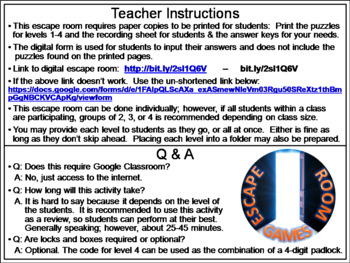# One Digit Subtraction Activity: Escape Room MathSubject
Resource Type
File Type

PDF

(1003 KB|14 pages)
Product Rating
Standards
• Product Description
• StandardsNEW

This breakout escape room is a fun way for students to test their skills with single digit subtraction. This escape room includes an optional digital form for students to input their answers. The puzzles will still need to be printed out to accompany the google form.

Contents:

♦ Teacher Instructions and FAQ

♦ 4 Puzzles for students to decode

♦ Student Recording Sheet and Teacher Answer Key

♦ Link to an optional, but recommended, digital breakout room

Check out the preview for more details!

Add and subtract within 20, demonstrating fluency for addition and subtraction within 10. Use strategies such as counting on; making ten (e.g., 8 + 6 = 8 + 2 + 4 = 10 + 4 = 14); decomposing a number leading to a ten (e.g., 13 - 4 = 13 - 3 - 1 = 10 - 1 = 9); using the relationship between addition and subtraction (e.g., knowing that 8 + 4 = 12, one knows 12 - 8 = 4); and creating equivalent but easier or known sums (e.g., adding 6 + 7 by creating the known equivalent 6 + 6 + 1 = 12 + 1 = 13).
Total Pages
14 pages
Included
Teaching Duration
N/A
Report this Resource to TpT
Reported resources will be reviewed by our team. Report this resource to let us know if this resource violates TpT’s content guidelines.